# Balancing Chemical Equations. Balancing redox equationsPage 2

#### WATCH ALL SLIDES

(b) NO2(g) + H2(g)  NH3(g) + H2O(g)

Answer: NO2(g) + 3 ½ H2(g)  NH3(g) + 2H2O(g)

(c) SO2(g) + O2(g)  SO3(g)

Answer: 2SO2(g) + O2(g)  2SO3(g)

Slide 6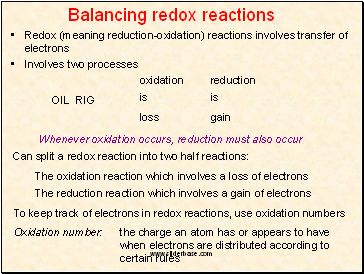## Balancing redox reactions

Redox (meaning reduction-oxidation) reactions involves transfer of electrons

Involves two processes

oxidation

is

loss

reduction

is

gain

Can split a redox reaction into two half reactions:

The oxidation reaction which involves a loss of electrons

The reduction reaction which involves a gain of electrons

To keep track of electrons in redox reactions, use oxidation numbers

Oxidation number: the charge an atom has or appears to have when electrons are distributed according to certain rules

OIL RIG

Whenever oxidation occurs, reduction must also occur

Slide 7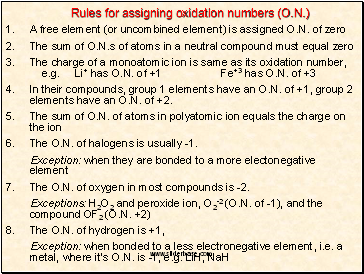## Rules for assigning oxidation numbers (O.N.)

A free element (or uncombined element) is assigned O.N. of zero

The sum of O.N.s of atoms in a neutral compound must equal zero

The charge of a monoatomic ion is same as its oxidation number, e.g. Li+ has O.N. of +1 Fe+3 has O.N. of +3

In their compounds, group 1 elements have an O.N. of +1, group 2 elements have an O.N. of +2.

The sum of O.N. of atoms in polyatomic ion equals the charge on the ion

The O.N. of halogens is usually -1.

Exception: when they are bonded to a more electonegative element

7. The O.N. of oxygen in most compounds is -2.

Exceptions: H2O2 and peroxide ion, O2-2 (O.N. of -1), and the compound OF2 (O.N. +2)

8. The O.N. of hydrogen is +1,

Exception: when bonded to a less electronegative element, i.e. a metal, where it’s O.N. is -1, e.g. LiH, NaH

Slide 8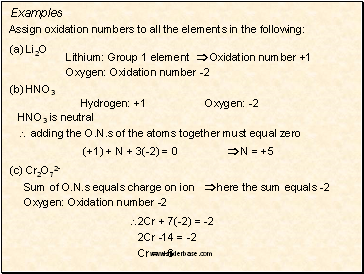Examples

Assign oxidation numbers to all the elements in the following:

(a) Li2O

Lithium: Group 1 element  Oxidation number +1

Oxygen: Oxidation number -2

(b) HNO3

Hydrogen: +1

Oxygen: -2

HNO3 is neutral

 adding the O.N.s of the atoms together must equal zero

(+1) + N + 3(-2) = 0  N = +5

(c) Cr2O72-

Sum of O.N.s equals charge on ion  here the sum equals -2

Oxygen: Oxidation number -2

2Cr + 7(-2) = -2

2Cr -14 = -2

Cr = +6

Slide 9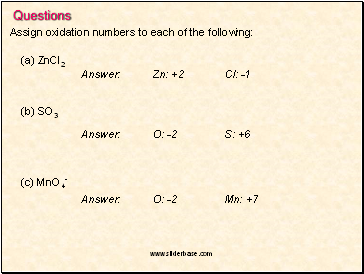Questions

Assign oxidation numbers to each of the following:

(a) ZnCl2

(b) SO3

(c) MnO4-

Slide 10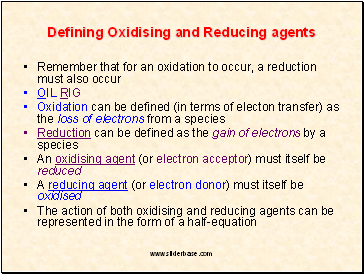## Defining Oxidising and Reducing agents

Remember that for an oxidation to occur, a reduction must also occur

Go to page:
1  2  3  4  5  6  7  8